# The 6th Annual Toscar Awards Red Carpet 2013

<p< < p=””><p< < p=””> </p< <>

After the Oscars were announced, the Toscars participants had only three weeks to make a short parody of the films nominated as the Best Picture of the Year, which screened at the world famous Egyptian Theatre, in Hollywood and critiqued by celebrity judges. The Awards the judges decided upon include ‘Best Whactor’, ‘Best Whactress’, ‘Best Film’ and more. The ceremony, organized by Brits in LA, is for parody sendups of the movies nominated for Best Picture.<p< < p=””> <p< < p=””> <p< < p=””> <p< < p=””> </p< <></p< <></p< <></p< <>

The 6th Annual Toscar Awards 2013 winner is Miserable Lesbians which won a record eight Golden Fists, including best film. <p< < p=””> <p< < p=””> <p< < p=””> <p< < p=””> </p< <></p< <></p< <></p< <>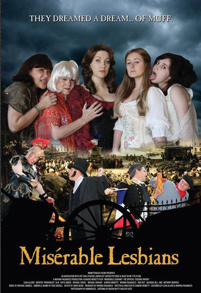<p< < p=””> </p< <>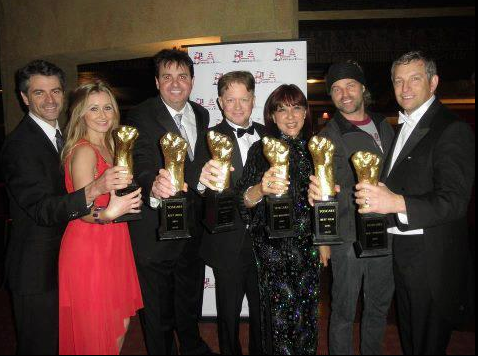<p< < p=””> </p< <>

<p< < p=””> <p< < p=””> </p< <></p< <>

Written and Directed by SANDRO MONETTI (winner best writer and director)
Produced by MARINA PAGANUCCI (winner best film – audience award-best film)
Composer : Michael Daniel
Starring: Leah Allers (winner best whactress)
Rob Pait
Natasha Sill (winner best suporting whactress)
Michael Enright (winner best supporting whactor)
Katie Amess
Kerstin Alm
Anthony Wemyss
Camera and sound; Reid Russell
Miserable Lesbians can be seen on You Tube Miserable Lesbians on YouTube
It is now being adapted for the stage and will be seen July 2013 in New York and August 2013 at the Edinburgh Fringe Festival. <p< < p=””> <p< < p=””> <p< < p=””> <p< < p=””> <p< < p=””> <p< < p=””> </p< <></p< <></p< <></p< <></p< <></p< <>

Other titles included:<p< < p=””> <p< < p=””> <p< < p=””> </p< <></p< <></p< <>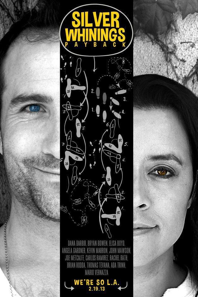<p< < p=””> <p< < p=””> </p< <></p< <>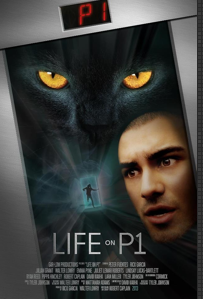<p< < p=””> <p< < p=””> </p< <></p< <>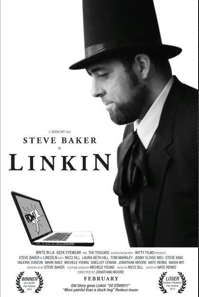<p< < p=””> <p< < p=””> </p< <></p< <><p< < p=””> <p< < p=””> <p< < p=””> <p< < p=””> </p< <></p< <></p< <></p< <>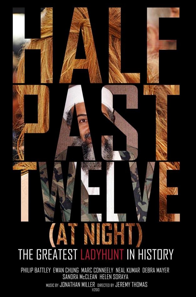<p< < p=””> <p< < p=””> </p< <></p< <><p< < p=””> <p< < p=””> <p< < p=””> </p< <></p< <></p< <><p< < p=””> <p< < p=””> </p< <></p< <>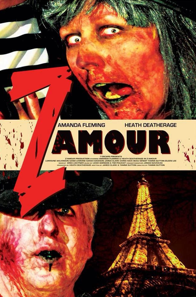<p< < p=””> <p< < p=””> </p< <></p< <>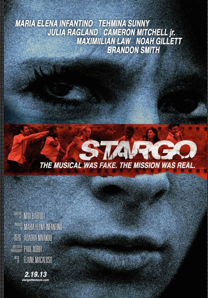<p< < p=””> </p< <>

.

Actor Eric Roberts headed a judging panel at the Egyptian Theatre. A stage version of “Miserable Lesbians” written-directed by Sandro Monetti is coming to New York’s 59E59 Theatre in July. Monetti said, “I told Hugh Jackman and Helena Bonham Carter about it. Hugh asked who was playing him, and Helena asked if she could be in it.” <p< < p=””> <p< < p=””> </p< <></p< <>

Celebrity Guests included: Doris Roberts (Everybody Loves Raymond), Joanne Whalley (The Borgias), Josh Sussman (Glee), Stephen Kramer Glickman (Glee), Iqbal Theba (Glee), Lauren Potter (Glee), Tristan Macmanus (Dancing with The Stars), Tony Denison (Closer), Brea Bee (Silver Linings Playbook), Stephanie Erb (True Blood), Jill Michele Melean (Mad TV), Erin Cahil (ABC’s Red Widow), Alice Amter (The Big Bang Theory), Shaun Parkes (The River and Davinchi’s Demons). Crystal Reed (MTV’s Teen Wolf), Kelly Hu (CW’s Arrow), Jeanine Mason (So You Think You Can Dance), Tia Carrere (Dancing With The Stars), James Scott (Days of Our Lives), Martin Samuel (Academy Award Nominee), Eve Stewart (Academy Award Nominee for Les Mis), Paco Delago (Academy Award Nominee for Les Mis), Claudio Miranda (Academy Award Nominee for Life of Pi), Craig Robert Young (NAVY NCIS), Kevin Buxbaum (Life of Pi Producer), Melanie Marr (Real Housewives of Beverly Hills), Jim Tavaré (Californication) , Mark Rhino Smith (Argo), Tehmina Sunny (Argo), Leilani Dowding (British Model), Kevin Buxbaum (Life of Pi Producer), Ben Stein (Family Guy), Will Brandt (Shock Value), Phaao Penghlish (Days of Our Lives), Greg Travis (Starship Troopers).<p< < p=””> <p< < p=””> <p< < p=””> <p< < p=””> </p< <></p< <></p< <></p< <>